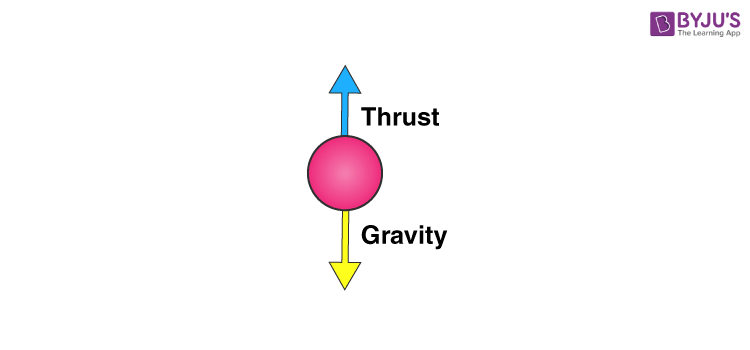Checkout JEE MAINS 2022 Question Paper Analysis : Checkout JEE MAINS 2022 Question Paper Analysis :

# Thrust And PressureYou may have noticed that when you submerge something in water it appears to weigh less. If you have gone swimming, you feel as though your weight has reduced. This is because any fluid applies an upward force on a body submerged in it. This force is called thrust. This force is in the opposite direction to the force of gravity. Hence, it reduces the total force acting downwards due to gravity on the object and you feel as though your weight has reduced.### Pressure

Pressure is defined as the force per unit area. If a force F is applied on a surface of area A, then the pressure P is defined as:

P =

$$\begin{array}{l} \frac {F}{A}\end{array}$$

As you blow up a balloon, it becomes harder to blow the balloon as it becomes bigger and bigger. This is because the air pressure inside the balloon is increasing. Pressure is expressed in N/m2 which is also called a Pascal (Pa).

In fluids this pressure is always perpendicular to the surface of an object. It is this pressure that is applied on an object submerged in water that forms the thrust force.

The pressure applied by the fluid is:

$$\begin{array}{l} Pressure \end{array}$$
=
$$\begin{array}{l} \frac {Thrust}{Area}\end{array}$$

Also,

$$\begin{array}{l} 1~Pascal ~(Pa) \end{array}$$
=
$$\begin{array}{l} \frac {1~Newton~(N)}{1~Metre~square~(m^2)}\end{array}$$

Or

$$\begin{array}{l} Thrust \end{array}$$
=
$$\begin{array}{l} Pressure~×~Area\end{array}$$

This pressure which produces a thrust on the object is the reason some objects float in water. It is also the reason why objects weigh less in water. To know why objects float or sink, see our article on the Archimedes Principle. Download BYJU’s-The Learning App to learn practically and interactively. We also have loads of fun learning videos and practice questions that you can do at your own pace. So go ahead and check it out in Byju’s.

## Frequently Asked Questions – FAQs

### What is thrust?

Thrust is the force acting normally on a surface. Its SI unit is Newton (N).Thrust is the force which moves an aircraft through the air. Thrust is used to overcome the drag of an aeroplane, and to overcome the weight of a rocket.

### What is pressure?

The force applied perpendicular to the surface of an object per unit area over which that force is distributed is known as pressure.

### What is buoyant force?

The buoyant force is the upward force exerted on an object wholly or partly immersed in a fluid. This upward force is also called Upthrust. Due to the buoyant force, a body submerged partially or fully in a fluid appears to lose its weight, i.e. appears to be lighter.

### What is Archimedes’ principle?

The upward buoyant force that is exerted on a body immersed in a fluid, whether partially or fully submerged, is equal to the weight of the fluid that the body displaces and acts in the upward direction at the centre of mass of the displaced fluid.

### Define pascal.

A pascal can be defined as a force of one newton applied over a surface area of a one-metre square.

Test your knowledge on Thrust Pressure

1. Nandana

Nice

3. Priyani

Excellent

4. Rashee Ghosh

Wonderful

5. piyush sharma

outstanding

6. Best Explanation